# PSAT Math : How to divide negative numbers

## Example Questions

### Example Question #41 : Integers

What is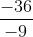?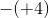45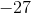Explanation:

A negative number divided by a negative number always results in a positive number.divided byequals. Since the answer is positive, the answer cannot beor any other negative number.

### Example Question #1 : Negative Numbers

Solve for: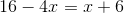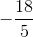Explanation:

Subtractfrom both sides: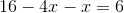, or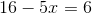Next, subtractfrom both sides: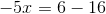, or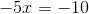Then, divide both sides by: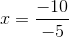Recall that division of a negative by a negative gives you a positive, therefore: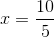or